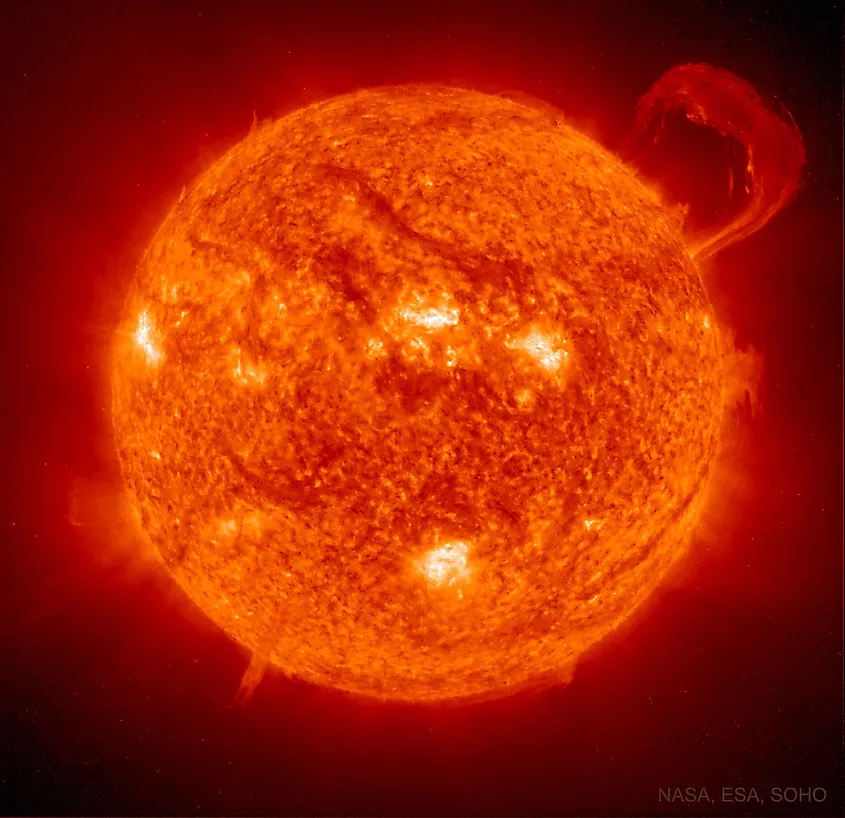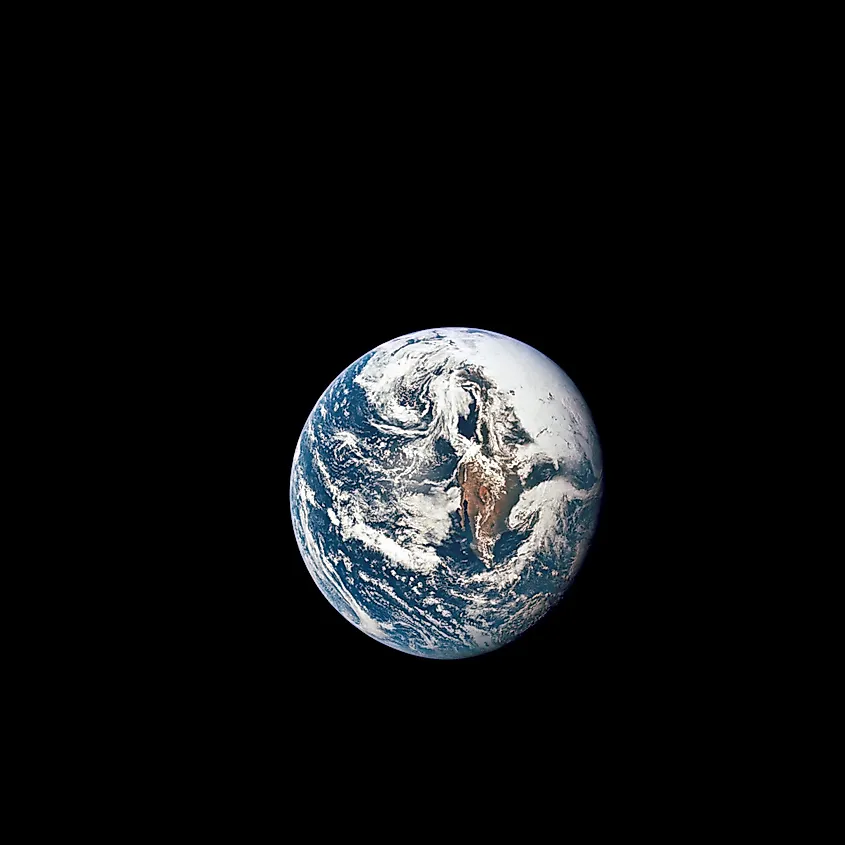# How Far Away Is The Sun?

The Earth orbits the sun at an average distance of 93-million miles (150-million kilometres). That distance is an average because the actual amount of distance between the Earth and sun changes as the Earth orbits the sun. Every object that orbits another object will have an elliptical shaped orbit. Although we may think of orbits as being circular, they are in fact elongated and appear more egg-shaped than circular.

## Maximum And Minimum DistanceThe sun is, on average, 93-million miles (150-million kilometres) away from the Earth. Image credit: NASA/ESA

When a planet is at its closest approach to its star, it is referred to as being at perihelion. For Earth, perihelion is the minimum amount of distance between it and the sun, which is about 91-million miles (147-million kilometres). The furthest distance is referred to as aphelion. When the Earth is at aphelion, it is 94.5-million miles (152-million kilometres) away from the sun. Perihelion and aphelion occur almost exactly six months apart. Perihelion occurs in early January while aphelion occurs in early July. That means that Earth is at its minimum distance during the coldest part of the year, while it is at its maximum distance during the hottest part of the year. Interestingly, it is not the distance between the Earth and sun that determines the seasons, but rather, it is the tilt of Earth’s axis. Although if the Earth were significantly closer or further away from the sun it would impact the Earth’s temperature, the eccentricity of Earth’s orbit is not extreme enough to have a noticeable impact on seasons.

## Astronomical UnitAn astronomical unit is defined as the average distance between the Earth and sun. Image credit: NASA

The average distance between the Earth and sun is also called an astronomical unit (AU). Since distances in space can be so vast, astronomers generally simplify units to make calculations easier and so that such large distances are easier to grasp. One astronomical unit is equal to 93-million miles (150-million kilometres). Using miles or kilometres as units usually results in gigantic numbers when dealing with space, and so astronomical units are a simple way of seeing how far away something is. For example, Neptune is 30 astronomical units away from the sun, or 30 times further from the sun than Earth.